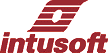MONTE, Monte Carlo Analysis: Before performing this analysis, (actual MONTE.SCP file), you must first set appropriate tolerance values on instance and model parameters using the part properties dialog for each part or class of parts. Then you need to select the number of lots and cases to run from the . Then make sure the Monte template is selected, and the radio button above the question mark is selected. The Data reduction should be set to Interactive and the Script checkbox must be checked. Then Select one of the appropriate Test Configurations and press Simulate Selections. Progress will be shown in the IsSpice output window. You can define the measurement results you want to view either before or after running the analysis. Measurements defined before running the analysis will be recorded in the database and can be view using the button. The measurements are set up for 5 sigma high values. Use the Set Limits dialog if you are establishing measurement tolerances. The usual procedure is to leave the nominal unchanged and choose expand to pass with symmetry to setup a symmetrical tolerance band based on the Monte Carlo results. A new dialog in IntuScope can be activated after running a Monte Carlo analysis by pressing the button in the dialog. To plot the statistical results, you must have saved measurements in the "prob" plot. You can add to the ones there using the dialog. Selecting vectors and functions from the appropriate lists does this; then press the button. Incidentially, you can plot all cases of each vector by selecting a vector's x and y axis and pressing the button. The really neat stuff happens when you select a measurement from the "prob" plot and press the plot group buttons. You get histograms or cumulative probability plots scaled to your data set. Cumulative probability warps the x axis into "sigmas" based on a normal distribution. For small sample sizes, it is much easier to visualize the distribution. We also plot the best fit of a straight line through these data points. If the data is normally distributed, it will lay along this straight line. The slope of the line, or first polynomial coefficient is an estimate of the standard deviation. The rms error is shown, and if it's small compared to the standard deviation, you are probably looking at a normal distribution. Next there is a feature to isolate the data set that created each data point. Simply place cursor 0 on a data point and press the report button. It tells you which simulation produced the data and shows each parameters value and its sigma deviation. Using this feature, you can get an approximate separation of class members for cases that have bi-modal distributions. Then you can separate class members into several groups for more detailed investigation. Note: To place cursor 0 exactly on a data point, place it to the left of the point and with a blank accumulator, press the "0->Y" button in the Cursor control toolbar. The cursor advance to the next data point each time you press the button. Using the "Y<-0" marches the cursor backward along data points. Finally, you can substitute the values associated with one of the simulations back into the schematic to evaluate a "worst case" or re-center the design.